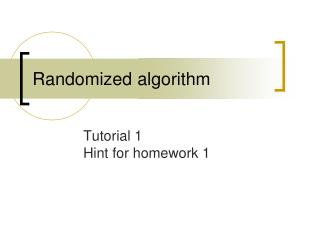# Randomized algorithm - PowerPoint PPT PresentationDownload PresentationRandomized algorithm

Randomized algorithmDownload Presentation## Randomized algorithm

- - - - - - - - - - - - - - - - - - - - - - - - - - - E N D - - - - - - - - - - - - - - - - - - - - - - - - - - -
##### Presentation Transcript

1. Randomized algorithm Tutorial 1Hint for homework 1

2. Outline • Lookup table problem (Exercise 1.18) • Convex function (Exercise 2.10) • Hint for homework 1

3. Lookup table problem

4. F:{0,…,n-1}→{0,…,m-1}. For any x and y with 0≦x, y≦n-1: F((x + y) mod n) = (F(x) + F(y)) mod m. Lookup table problem n=10, m=7

5. Lookup table problem • Now, someone has changed exactly 1/5 of the lookup table entries

6. Lookup table problem • (a) Given any input z, outputs the correct value F(z) with probability at least 1/2. (it needs to work for every value) • Pick a x, uniformly at random from [0,…,n-1]. • Compute y =z-x mod n. • output (F(x)+F(y)) mod m as our computed value for F(z).

7. Lookup table problem • Pr (the output F(z) is correct) ≧Pr (F(x) and F(y) are not modified) ＝1-Pr (F(x) or F(y) are modified) ≧1-(Pr (F(x) is modified) + Pr (F(y) is modified)) ≧1-(1/5 + 1/5) = 3/5

8. Lookup table problem • (b) Can you improve the probability of returning the correct value with repeating your algorithm three times? • Pick three values (with replacement) of y1, y2, y3 as y values. • compute the corresponding values of F(z1),F(z2),F(z3). • If two or more of the F(z) values are equal, we output such value. Otherwise, we output any one of these values.

9. Lookup table problem • Pr (the output F(z) is correct) ≧Pr (at least two of the F(zi),F(z2),F(z3) are correct) ＝Pr (exactly two of the F(z1),F(z2),F(z3) are correct)+Pr (all F(z1),F(z2),F(z3) are correct) ≧3*3/5*3/5*2/5+3/5*3/5*3/5 ＝54/125+27/125＝81/125

10. Convex function

11. Convex function • Show by induction that if f:R→R is convex then, for any x1, x2, …, xn and y1, y2, …, yn with

12. Convex function (y1x1+y2x2,y1f(x1)+y2f(x2)) x2 x1

13. Convex function

14. Convex function • Use former inequality to prove that if f:R→R is convex then E[f(X)] ≧f(E[X])

15. Hint for homework 1

16. Homework 1-1 step 1 step 2 What is the probability? ：a ：c Box 1 Box 2 ：b ：d

17. Homework 1-1 • Hint: There are two cases • The ball from one to the other is black. • The ball from one to the other is white.

18. Homework 1-2 step 2 step 1 Pr( )=1/3 ：a ：b The probability we get a white ball at the first time is a/a+b.

19. Homework 1-3 • Give an example of three random events X, Y, Z for which any pair are independent but all three are not mutually independent. • Hint: Two dices with X=sum is even. You may use to check your answer.

20. Homework 1-4 • There can be at most n(n-1)/2 distinct min-cut sets. • Hint: [Theorem 1.8] The algorithm outputs a min-cut set with probability at least 2/n(n-1).

21. Homework 1-5 20 • Hint: Indicator variable/linearity of expectation.

22. Homework 1-6 • (a) What is the probability that X = Y ? • (b) What is E[max(X,Y )]? • min(X,Y) is the random variable denoting the number of steps you see the first head. X+Y-min(X,Y) = max(X,Y). • Memory-less property of geometric random variable.

23. 1 2 m m+1 m+2 n Homework 1-7 • First, we give everyone a number card. The number card means the order of interview. • Choose the best grade from 1 to m : A. • If someone after m better than A, accept him. Otherwise, we choose the last one.

24. Homework 1-7 • Sometimes, we may not get the best candidate. • The best one’s number card is less than m+1. • A, the best grade we chose from 1 to m, is not too good. • What is a “nice” m? • The second best candidate is in 1~m.